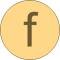# Helmholtz_pT

Function to calculate analytic derivatives for computing d and t given p and t# Syntax

nderivs = Helmholtz_pT(f)

# Inputs (1)

f Type: HelmholtzDerivs Description: Dimensionless derivatives of Helmholtz function

# Outputs (1)

nderivs Type: NewtonDerivatives_pT Description: Derivatives for Newton iteration to compute d and t from p and t